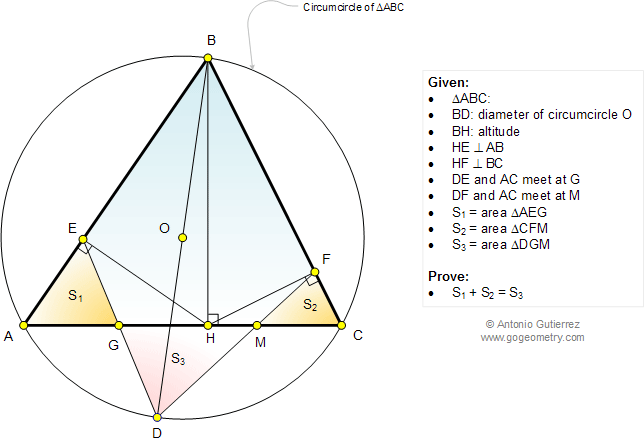# Infographic Geometry Problem 1058: Triangle, Sum of the Areas, Altitude, Perpendicular, Circumcircle, Circle, Diameter. Level: High School, SAT Prep, College, Mathematics Education

< PREVIOUS PROBLEM  |  NEXT PROBLEM >

 In a triangle ABC, BH is the altitude and BD is a diameter of the circumcircle O (see the figure below). HE and HF are perpendicular to AB and BC at E and F, respectively. DE and DF meet AC at G and M, respectively. If S1 = area of triangle AEG, S2= area of triangle CFM, and S3 = area of triangle DGM, prove that S1 + S2 = S3.Home | Search | Geometry | Problems | All Problems | Open Problems | Visual Index | 10 Problems | 1051-1060 | Triangle | Area | Triangle Area | Altitude | Perpendicular lines | Circle | Circumcircle | Diameter | Email | by Antonio Gutierrez Add or view a solution to the problem 1058 Last updated: Dec 2, 2014